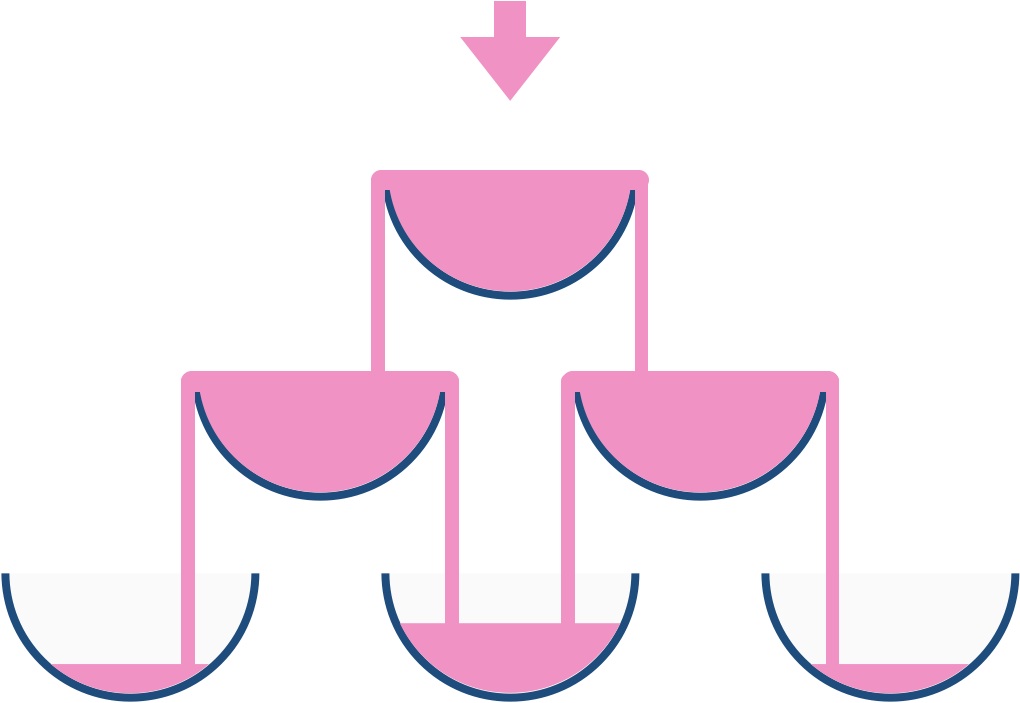GabrielxD

• 累计撰写 673 篇文章
• 累计创建 128 个标签
• 累计收到 15 条评论

### 目 录CONTENT# 【模拟, 动态规划】香槟塔2022-11-20 / 0 评论 / 0 点赞 / 179 阅读 / 795 字

## 题目

799. 香槟塔示例 1:



输入: poured = 100000009, query_row = 33, query_glass = 17



• 0 <= poured <= 10^9
• 0 <= query_glass <= query_row < 100

## 解题

### 方法一：动态规划 模拟

#### 代码

class Solution {
public double champagneTower(int poured, int query_row, int query_glass) {
double[][] tower = new double[query_row + 1][query_row + 1];
tower = poured;

for (int row = 0; row < query_row; row++) {
for (int col = 0; col <= row; col++) {
if (tower[row][col] <= 1) continue;
tower[row + 1][col] += (tower[row][col] - 1) / 2;
tower[row + 1][col + 1] += (tower[row][col] - 1) / 2;
}
}

return Math.min(tower[query_row][query_glass], 1);
}
}

class Solution {
public:
double champagneTower(int poured, int query_row, int query_glass) {
vector<vector<double>> dp(query_row + 1, vector<double>(query_row + 1));
dp = poured;
for (int i = 0; i < query_row; ++i) {
for (int j = 0; j <= i; ++j) {
if (dp[i][j] > 1) {
--dp[i][j];
dp[i + 1][j] += dp[i][j] / 2;
dp[i + 1][j + 1] += dp[i][j] / 2;
}
}
}
return min(1.0, dp[query_row][query_glass]);
}
};

0